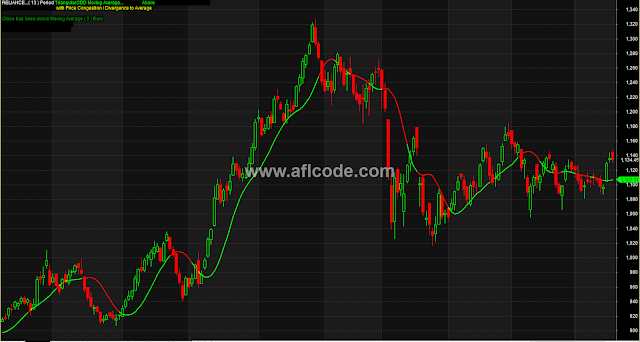### Change Of Trend Detector With Triangular Moving AverageChange Of Trend Detector With Triangular Moving Average
```//www.aflcode.com

Odd=13;//enter Odd numbers only
CoefOdd=round(Odd/2);

Even=12;//enter Even numbers only
Coefeven=Even/2;
Coefeven2=Coefeven+1;

CongestionPercent=2.8;/*Set % above/below Moving average for congestion / sideways market*/

TriangularOdd=MA(MA(C,CoefOdd),CoefOdd);
TriangularEven=MA(MA(C,Coefeven),Coefeven2);

finalMov_avg=IIf(Odd > even,triangularOdd,TriangularEven);

Color=colorBrightGreen;//select Moving average line color
tickercolor=colorBlack;//select price color

Plot(finalMov_avg,"",IIf(C < finalmov_avg,colorRed,Color),styleLine|styleThick);
Plot(C,"",tickercolor,styleCandle);

Title=Name()+"..."+"( "+WriteIf(Odd > even,WriteVal(Odd,1),WriteVal(even,1))+" ) Period "+EncodeColor(Color)+"Triangular"+WriteIf(Odd > even,"ODD","EVEN")+" Moving Average"+"..."+EncodeColor(colorBlack)+ WriteIf(C < finalMov_avg,"Close is "+EncodeColor(colorRed)+"Below"+EncodeColor(colorBlack)+" Moving Average by ","Close is"+EncodeColor(colorBrightGreen)+" Above"+EncodeColor(colorBlack)+" Moving Average by ")+"("+WriteVal(((C/finalMov_avg)-1)*100,1.1)+"% )"+"\n"+WriteIf(finalmov_avg-Ref(finalmov_avg,-1)>0," Slope Of Average is UP : ","Slope Of Average is DOWN :")+WriteIf((((C/finalMov_avg)-1)*100 <= CongestionPercent AND ((C/finalMov_avg)-1)*100 >= -CongestionPercent),EncodeColor(colorYellow)+" with Price Congestion / Divergence to Average ","")+"\n"+WriteIf(Ref(C,-1) < Ref(finalmov_avg,-1) AND C > finalmov_avg,EncodeColor(colorGreen)+"Possible Change in Trend From Down to Up"+"\n"+" OR Short Term Correction of Previous Trend",WriteIf(Ref(C,-1) > Ref(finalmov_avg,-1) AND C < finalmov_avg,EncodeColor(colorRed)+"Possible Change in Trend From Up to Down "+"\n"+" OR Short Term Correction to Previous Trend",""))+"\n"+WriteIf(C > finalmov_avg,EncodeColor(colorGreen)+"Close has been above Moving Average ( "+WriteVal(BarsSince(C < finalmov_avg),1)+" ) Bars",EncodeColor(colorRed)+"Close has been Below Moving Average ( "+WriteVal(BarsSince(C > finalmov_avg),1)+" ) Bars")+"\n"+EncodeColor(colorBlack)+"The average # of Bars Above ( "+WriteVal(round(Cum(BarsSince(C < finalmov_avg)/Cum(1))),1)+" )"+"\n"+"The average # of Bars Below ( "+WriteVal(round(Cum(BarsSince(C > finalmov_avg)/Cum(1))),1)+" )";
```
Previous
Next Post »